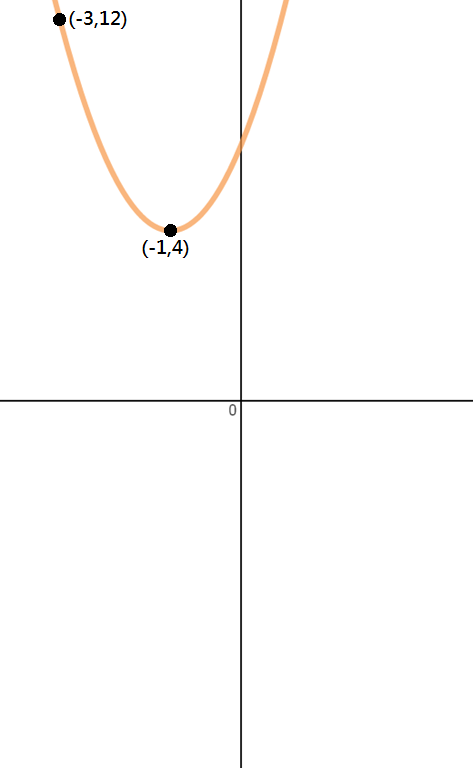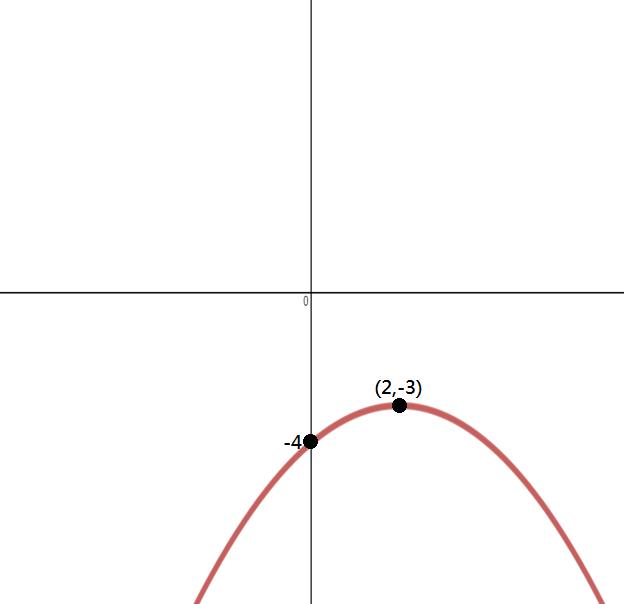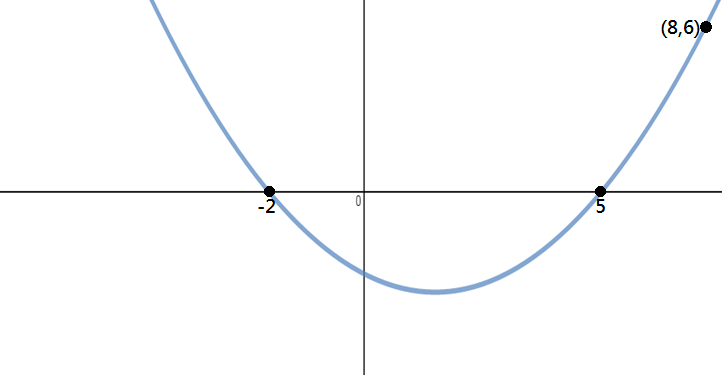# Finding the quadratic functions for given parabolas#### Everything You Need in One Place

Homework problems? Exam preparation? Trying to grasp a concept or just brushing up the basics? Our extensive help & practice library have got you covered.#### Learn and Practice With Ease

Our proven video lessons ease you through problems quickly, and you get tonnes of friendly practice on questions that trip students up on tests and finals.#### Instant and Unlimited Help

Our personalized learning platform enables you to instantly find the exact walkthrough to your specific type of question. Activate unlimited help now!

0/0
0/4
##### Examples
###### Lessons
1. Determine the equation of the parabola.

1.2.3.4. vertex: $(-3, -2)$
x-intercept: 1
###### Topic Notes
In the last lesson, we learned how to draw a parabola from its function. This lesson, we will do the opposite. We will learn how to find the quadratic function when we are given the graph of a parabola.

## Finding the Quadratic Functions for Given Parabolas

At this point, you should be relatively familiar with what parabolas are and what they look like. But, to make sure you're up to speed, a parabola is a type of U-Shaped curve that is formed from equations that include the term $x^{2}$. Oftentimes, the general formula of a quadratic equation is written as: $y = (x-h)^{2} + k$. Below is an image of the most simple quadratic expression we can graph, $y = x^{2}$.

There are so many different types of problems you can be asked with regards to quadratic equations. In this article, the focus will be placed upon how we can develop a quadratic equation from a quadratic graph using a couple different methods. But, before we get into these types of problems, take a moment to play around with quadratic expressions on this wonderful online graphing calculator here. The more comfortable you are with quadratic graphs and expressions, the easier this topic will be!

Now let's get into solving problems with this knowledge, namely, how to find the equation of a parabola!

## How to Find a Quadratic Equation from a Graph:

In order to find a quadratic equation from a graph, there are two simple methods one can employ: using 2 points, or using 3 points.

1) Find Quadratic Equation from 2 Points

In order to find a quadratic equation from a graph using only 2 points, one of those points must be the vertex. With the vertex and one other point, we can sub these coordinates into what is called the "vertex form" and then solve for our equation. The vertex formula is as follows, where (d,f) is the vertex point and (x,y) is the other point:

$(y \pm d) = a(x \pm f)^{2}$

Vertex form can also be written in its more "proper" form, as:

$y = a(x \pm f)^{2} \mp d$

Using this formula, all we need to do is sub in the vertex and the other point, solve for a, and then rewrite our final equation. The best way to become comfortable with using this form is to do an example problem with it.

Example:

Determine the equation of the parabola shown in the image below.

Step 1: Identify Points

Since we are only given two points in this problem, the vertex and another point, we must use vertex form to solve this question.

Given the information from the graph, we can determine the quadratic equation using the points of the vertex, (-1,4), and the point on the parabola, (-3,12).

Step 2: Sub Points Into Vertex Form and Solve for "a"

Now all we have to do is sub in our two points into the vertex formula and solve for "a" to have all the information to write our final quadratic equation. Recall vertex form:

$(y \pm d) = a(x \pm f)^{2}$

Using the coordinates of our vertex:

$y = 4$

$(y - 4) = 0$

$x = -1$

$(x + 1) = 0$

$(y - 4) = a(x + 1)^{2}$

Next, we have to solve for the value of "a" using the point (-3,12):

$(12 - 4) = a(-3 + 1)^{2}$

$8 = a(-2)^{2}$

$8 = 4a$

$a = 2$

Step 3: Write Out Quadratic Equation

After solving for "a", we now have all of the information we need to write out our final answer.

$y - 4 = 2(x + 1)^{2}$

And then, in proper vertex form of a parabola, our final answer is:

$y = 2(x + 1)^{2} + 4$

That completes the lesson on vertex form and how to find a quadratic equation from 2 points! If you want to refresh your memory on the related topics such as, how to solve quadratic expressions in vertex form, how to convert a regular quadratic equation from standard form to vertex form by completing the square, and how to use vertex formula, make sure to check out our lessons.

2) Find Quadratic Equation from 3 Points

In some instances, we won't be so lucky as to be given the point on the vertex. If that's the case, we can no longer find the quadratic expression using just two points, and need to do something a little different. In the case that we are given information about the x-intercepts of a parabola, as well as one other point, we can find the quadratic equation using an equation that is called "factored form". The general equation for the factored form formula is as follows, with b and c being the x-coordinate values of the x-intercepts:

$y = a(x - b)(x - c)$

Using this formula, all we need to do is sub in the x-coordinates of the x-intercepts, another point, and then solve for a so we can write out our final answer. Again, the best way to get comfortable with this form of quadratic equations is to do an example problem.

Example:

Determine the equation of the parabola shown in the image below:

Step 1: Identify Points

Since we are given three points in this problem, the x-intercepts and another point, we can use factored form to solve this question.

From the graph, we can see that the x-intercepts are -2 and 5, and the point on the parabola is (8,6).

Step 2: Sub Points Into Vertex Form and Solve for "a"

Now all we have to do is sub in our values into the factored form formula and solve for "a" to have all the information to write our final quadratic equation. Recall factored form:

$y = a(x - b)(x - c)$

Using the coordinates of the x-intercepts:

$x$-intercept = $-2$

$x = -2$

$(x + 2) = 0$

$x$-intercept = $5$

$x = 5$

$(x - 5) = 0$

$y = (x + 2)(x - 5)$

Next, we can use the point on the parabola (8,6) to solve for "a":

$6 = a(8 + 2)(8 - 5)$

$6 = a(10)(3)$

$6 = 30a$

$a = \frac{1}{5}$

Step 3: Write Out Quadratic Equation

After solving for "a", we now have all of the information we need to write out our final answer.

$y = \frac{1}{5} (x + 2)(x - 5)$

And that's all there is to it! Those are the two most important methods for finding a quadratic function from a given parabola. For further study into quadratic functions and their graphs, check out these useful videos dealing with the discriminant, graphing quadratic inequalities , and conic sections.